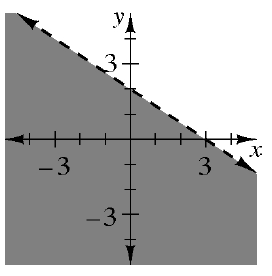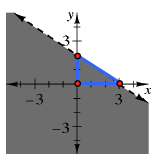Home > CCAA8 > Chapter cca10 > Lesson cca10.2.1 > Problem10-31

10-31.Write the inequality represented by the graph at right. Homework Help ✎

Use a slope triangle to find the slope of the line.

$\text{Slope} = -\frac{2}{3}$

Find the $y$-intercept on the graph. $(0,2)$Now substitute the slope and the $y$-intercept into the Slope-Intercept form of a line. $y=mx+b$

$\textit{y}=-\frac{2}{3}+2$

Replace the '$=$' with a '$<$' sign, as the graph has the portion below the line shaded.

$y<-\frac{2}{3}x+2$